1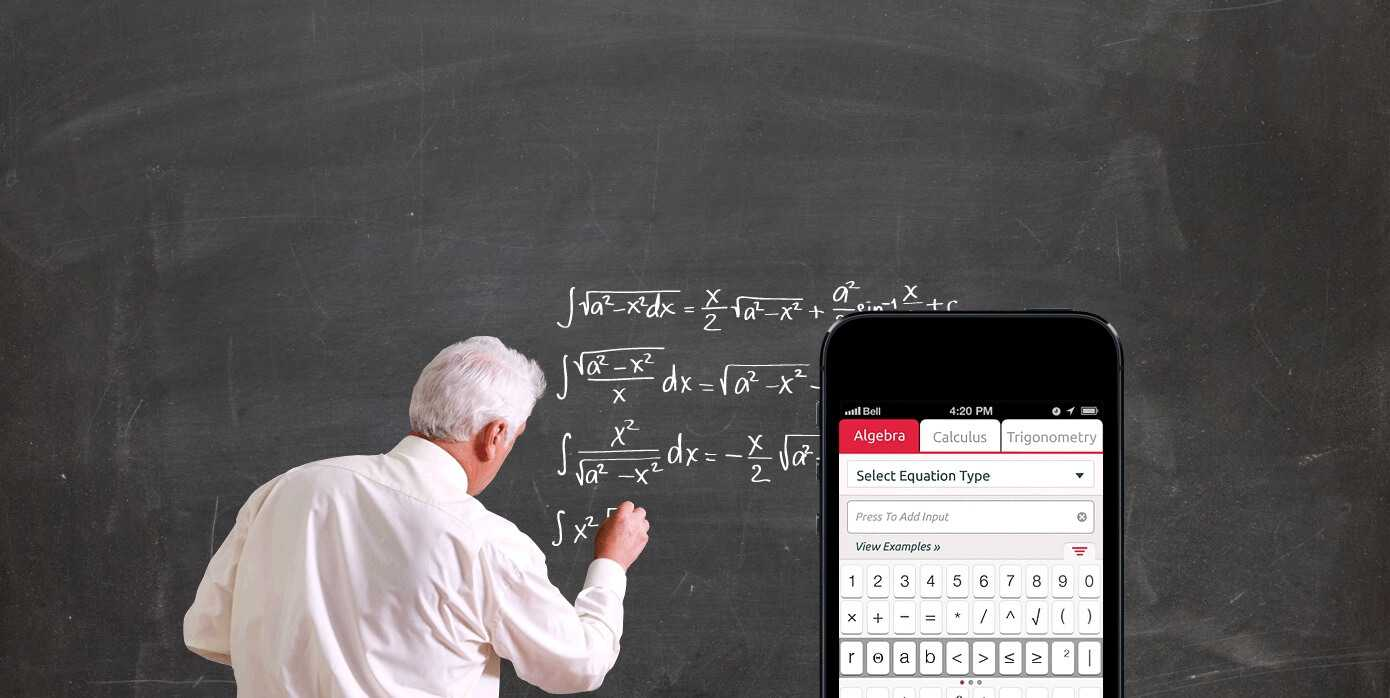# Symbolab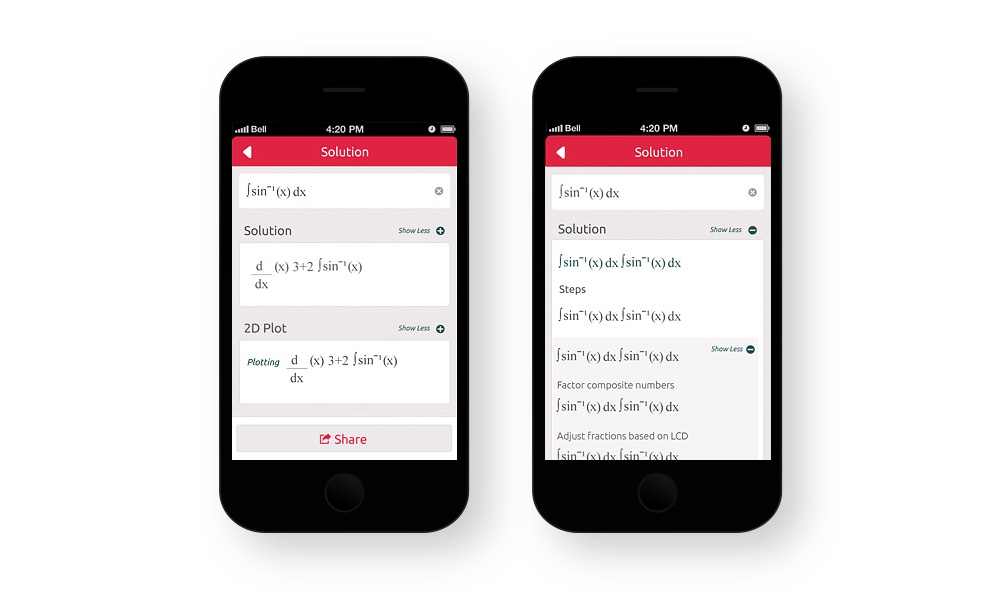Brief

An Israeli-based start-up team approached us with the idea to create a PhoneGap application for solving scientific equations. The application would combine two functions: semantic web search engine for math and science and maths problem solver.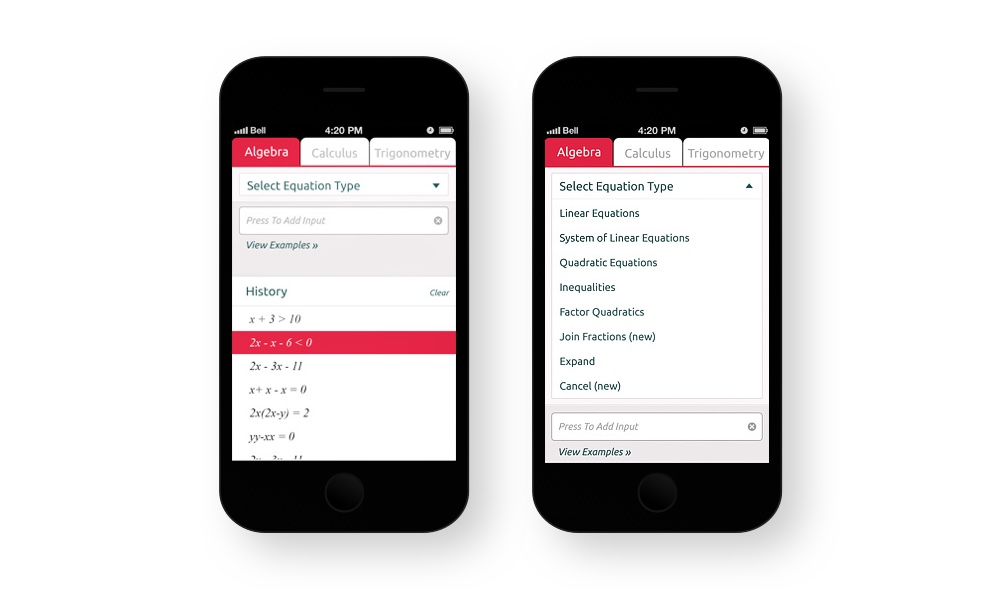Solution

As the system would work with large volumes of maths symbols and data, using pure HTML would be unreasonable. So we resorted to LATEX – a specific document markup language for displaying formulas.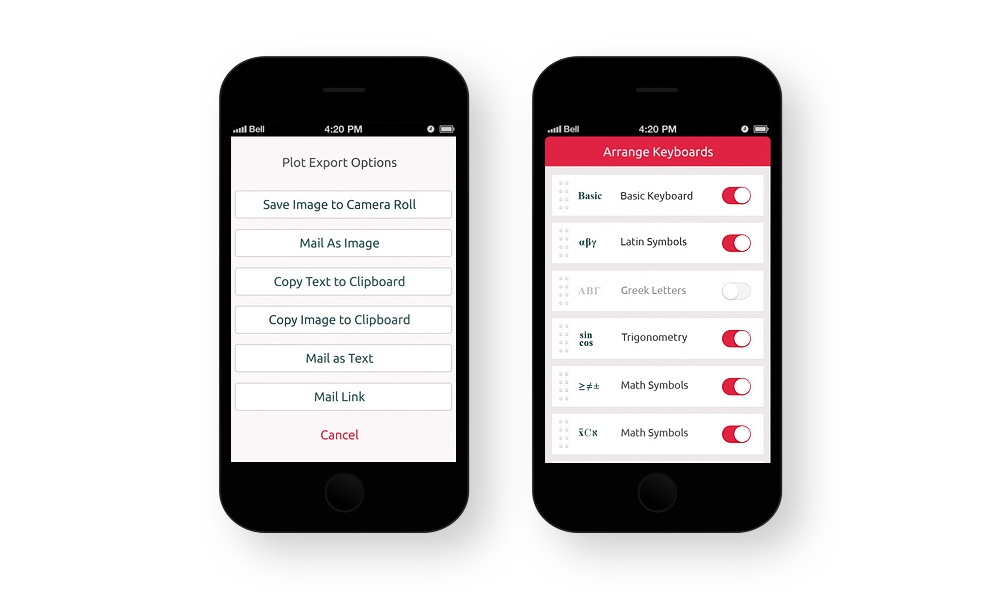Result

Symbolab is a PhoneGap application available for iOS and Android. It allows users to search and solve equations, formulas and expressions using mathematical symbols and scientific notations as well as text. Symbolab solves maths problems. It provides automated step by step solutions to algebraic and calculus topics covering from middle school maths through to college maths. Symbolab offers a wealth of maths calculators including: equations calculator, simultaneous equations calculator, inequalities calculator, integral calculator, derivative calculator, limits calculator, tangent line calculator, trigonometric equations calculator and more. This product can be widely used by students, engineers and scientists.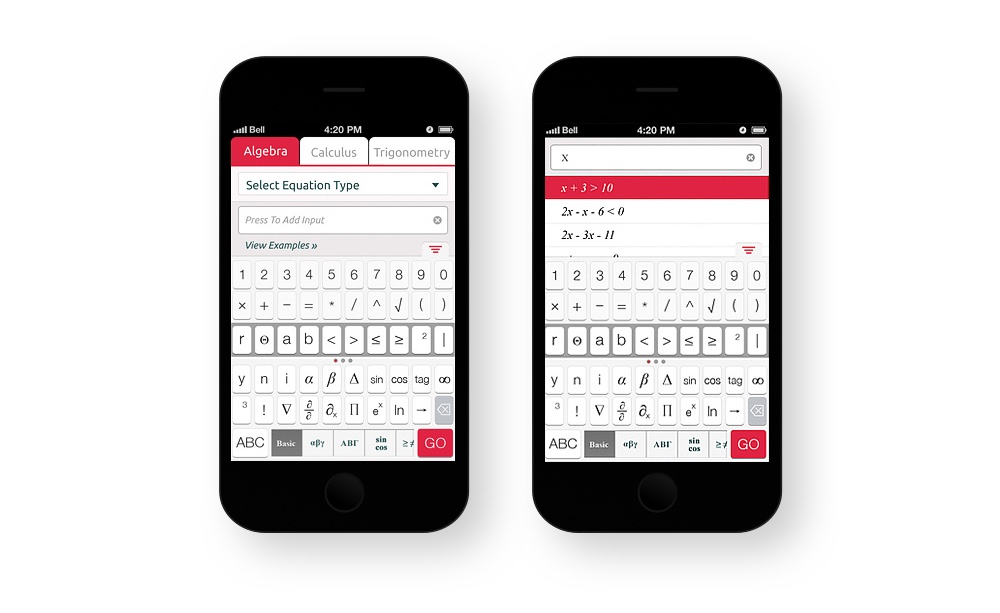nextEducation software
Couch callclose
Get in touch
Do you agree to the personal data processing?close
Thank you very much.

Your registration to the webinar on the 27th of September at 2 p.m. BST was successfuly completed.
We will send you a reminder on the day before the event.
Magora team
Registration for a webinar